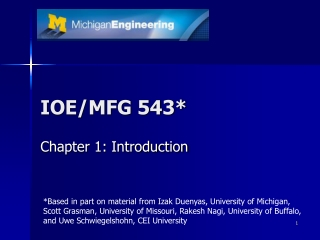DownloadDownload PresentationIOE/MFG 543*

# IOE/MFG 543*

Download Presentation## IOE/MFG 543*

- - - - - - - - - - - - - - - - - - - - - - - - - - - E N D - - - - - - - - - - - - - - - - - - - - - - - - - - -
##### Presentation Transcript

1. IOE/MFG 543* Chapter 1: Introduction *Based in part on material from Izak Duenyas, University of Michigan, Scott Grasman, University of Missouri, Rakesh Nagi, University of Buffalo, and Uwe Schwiegelshohn, CEI University

2. Scheduling • Allocation of scarce resources to tasks over time • Decision-making process with the goal of optimizing an objective (or multiple objectives)

3. Elements • Resources • Machines, runways, construction crews, CPUs • Tasks • Operations in a production process, take-offs and landings, stages in a construction project, execution of computer programs • Objectives • Minimizing last completion time • Maximize number of tasks completed on time

4. Examples • Paper bag factory • Gate assignments at an airport • Scheduling tasks in a CPU • What are the resources (machines), tasks (jobs), and objectives?

5. minimize SwjCjwhere Cj is the completion time of job j, pj is the processing time of job j, wj is the weight (priority) of job j 5 job single machine exercise

6. IOE/MFG 543 Chapter 2. (Sections 2.1-2.2)Deterministic models: Preliminaries

7. Jobs and machines • Data (assumed to be given)

8. Describing a scheduling problem || Machine environment Objective (to be minimized) Process characteristics and constraints

9. Machine environment a • Single machine and machines in parallel

10. Machine environment a (2) • Machines in series

11. Processing characteristics and constraints b

12. Processing characteristics and constraints b (2)

13. Objectives g • Performance measures of individual jobs

14. Objectives g (2) • Functions to be minimized

15. Regular objective functions • Regular objective functions • non-decreasing in C1,…,Cn • most objective functions considered in this class are regular • Non-regular objective functions • Example: Σwj' Ej+ Σwj'' Tj • Much harder to solve!

16. IOE/MFG 543 Appendix C. Complexity terminology

17. What does complexity mean? • Complexity is an indication of how much computation is required to solve a problem • Significance of the complexity of a scheduling problem • Does an efficient algorithm for solving the problem exist? • Worst case analysis

18. Problems and instances • A problem is the generic description of a problem • An instance refers to a problem with a given set of data • The size of an instance refers to the length of the data string necessary to specify the instance (on a computer) • In this class the size of an instance is usually measured in the number of jobs n

19. Measure of computation • Turing machine • an abstract representation of a computing device • In this class • Typical computation steps • comparison • multiplication • addition • other data manipulation

20. Order relation • An algorithm is an O(g(n)) algorithm if there exist a constant c>0, a function g(n), and an integer n0>0 such that the maximum number of iterations f(n) needed to find an optimal solution satisfies • If O(nk) for some finite k then polynomial time • O(n!) and O(4n) are not polynomial time

21. Optimization vs. decision problems • A decision problem • A question that requires either a “yes” or “no” answer • For every optimization problem there is an associated decision problem • For a given number z, is there a schedule x such that f(x)≤z?

22. The classes P and NP • Class P • The class P contains all decision problems for which there exists a Turing machine algorithm that leads to the right yes-no answer in a number of steps bounded by a polynomial in the length of the encoding

23. The classes P and NP (2) • Class NP • The class NP contains all decision problems for which the correct answer, given a proper clue, can be verified by a Turing machine in a number of steps bounded by a polynomial in the length of the encoding

24. Problem reduction • Problem P polynomially reduces to problem P’ if a polynomial time algorithm for P’ implies a polynomial time algorithm for problem P • Denoted P  P’ • P’ is at least as hard as P

25. The classes NP-hard and NP-complete • Class NP-hard • A problem P is called NP-hard if the entire class NP polynomially reduces to problem P • Problem P is at least as hard as all the problems in NP • Class NP-complete (not in the textbook) • A problem P is called NP-complete if it is both in classes NP and NP–hard

26. Pseudopolynomial algorithms • Polynomial time algorithms exist for some NP-hard problems under the appropriate encoding of the problem data • Such problems are referred to as NP-hard in the ordinary sense and the algorithms are called pseudopolynomial • Problem P is called strongly NP-hard if a pseudopolynomial algorithm for it does not exist

27. Some NP-hard problems • NP-hard in the ordinary sense • PARTITION • Strongly NP-hard • SATISFIABILITY • 3-PARTITION • HAMILTIONIAN CIRCUIT • CLIQUE